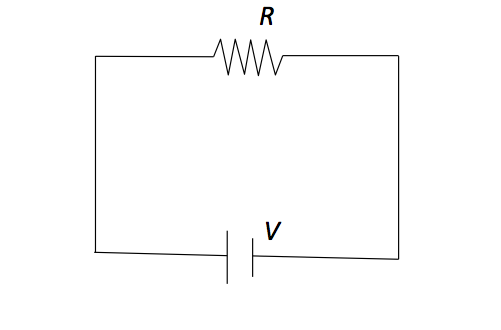Classical Mechanics

# Configurational entropy (qualitative)In the above circuit, the voltage across the resistor $R = 13 \ \Omega$ is $V = 0 \text{ V}.$ If the voltage $V$ is suddenly increased to $V' = 13 \text{ V},$ what has happened to the entropy of the system of electrons in the circuit?

If a warm object contacts a cold object, in which direction will the heat flow?

$100$ air particles are flying around a room. Suddenly, all the particles are moved to one side of the room. What has happened to the entropy of the system?

If we put a cup of water into a freezer, what will happen to its entropy?

If ice cream is melted in a warm room, what happens to the entropy of the system?

×